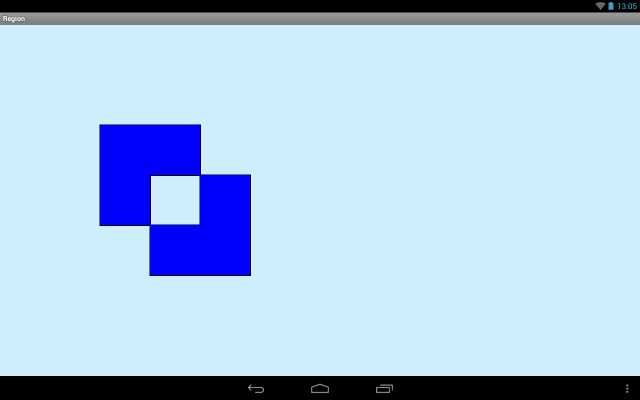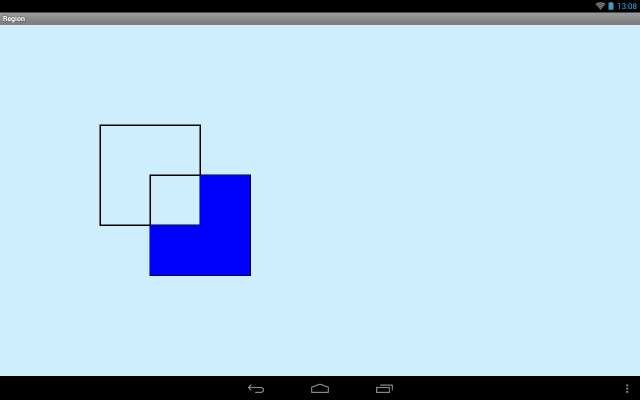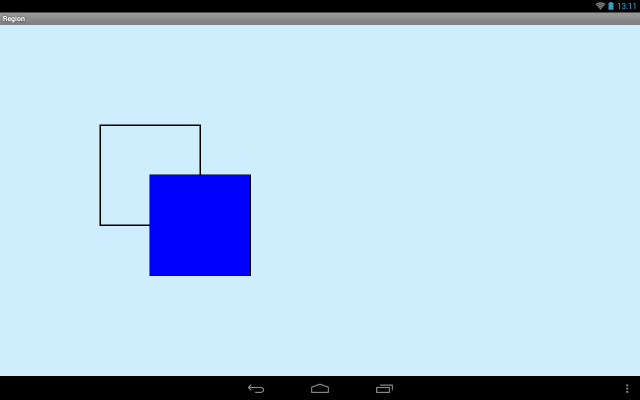In this lesson:

– we use Region

Region is an object that allows us to combine several shapes into one, using different modes: merging, intersection, etc. The words will be difficult to explain in detail, so let’s take an example.

Let’s create a project:

Project name: P1471_Region
Build Target: Android 2.3.3
Application name: Region
Package name: ru.startandroid.develop.p1471region
Create Activity: MainActivity

MainActivity.java:

```package ru.startandroid.develop.p1471region;

import android.app.Activity;
import android.content.Context;
import android.graphics.Canvas;
import android.graphics.Color;
import android.graphics.Paint;
import android.graphics.Path;
import android.graphics.Rect;
import android.graphics.Region;
import android.graphics.RegionIterator;
import android.os.Bundle;
import android.view.View;

public class MainActivity extends Activity {

@Override
protected void onCreate(Bundle savedInstanceState) {
super.onCreate(savedInstanceState);
setContentView(new DrawView(this));
}

class DrawView extends View {

Paint p;
Rect rect1;
Rect rect2;
Region region;
RegionIterator iterator;
Path path;

Region.Op op = Region.Op.UNION;

public DrawView(Context context) {
super(context);
p = new Paint();
p.setStrokeWidth(3);

// прямоугольники
rect1 = new Rect(200,200,400,400);
rect2 = new Rect(300,300,500,500);

// создание региона
region = new Region();
region.set(rect1);
region.op(rect2, op);

// создание path из региона
path = region.getBoundaryPath();
}

@Override
protected void onDraw(Canvas canvas) {
canvas.drawARGB(80, 102, 204, 255);

// контуры прямоугольников
p.setStyle(Paint.Style.STROKE);
p.setColor(Color.BLACK);
canvas.drawRect(rect1, p);
canvas.drawRect(rect2, p);

// path
p.setStyle(Paint.Style.FILL);
p.setColor(Color.BLUE);
canvas.drawPath(path, p);

}
}
}```

In the constructor DrawView we create objects. In our example, two rectangles rect1 and rect2 will participate. As can be seen from their coordinates, they intersect. Next, create a region and set it to the first rectangle (rect). The region now consists of one rectangle. To add extra rectangles, you must use the op method. Add a second rectangle (rect2) and specify the Region.Op.UNION mode (op variable).

If UNION mode is used when adding a new rectangle to a region, then the resulting region will be a union of the area of ​​the current region and a rectangle added. In our case, the region consisted of the first rectangle, so the result of adding the second would be to combine the areas of the first and second rectangles.

The getBoundaryPath method is used to get the final region of the region into a Path object so that the result of the merge can be drawn.

In the method onDraw we first draw the outline of the rectangles in black. Then in blue with fill we draw a path that represents the final region of the region.

result:We see that the region is a union of two rectangles. We were merged because we were using UNION mode.

We have considered one mode of addition and only six of them. To do this, our code uses the op variable:

`Region.Op op = Region.Op.UNION;`

Now here is the UNION value. Just change it to the modes we consider below.

Remember that the region first contains the first rectangle. And we add the second rectangle using a certain mode.

XORRegion summary area: The areas of both rectangles except their intersection.

DIFFERENCEArea summary area: The area of ​​the first rectangle except for intersection with the second rectangle.

REVERSE_DIFFERENCEArea summary area: The area of ​​the second rectangle except for intersection with the first.

INTERSECTRegion summary area: the intersection of both rectangles

REPLACEFinal Region Area: Second Rectangle. the content of the region has been replaced by a second rectangle.

In the help, you can see that the op method has several options, but the whole point is the same everywhere – adding a rectangle or an entire region to the current region using modes.

### RegionIterator

The total area of ​​a region can be divided into a set of disjoint rectangles. To do this, use RegionIterator – the region iterator. When you create, you specify the region and use the method next to select the rectangles that make up the region.

For the sake of interest, post the log to the iterator loop and print (Rect.toShortString) the coordinates of the regions that make up the region in the examples above. You will see how the iterator splits the region into disjoint rectangles.

In the case of UNION, for example, the log will be as follows:

rect = [200,200][400,300]
rect = [200,300][500,400]
rect = [300,400][500,500]

### Other methods

Let’s look at some more useful regional methods.

contains – allows you to determine whether a specified point is in a region

getBounds – will return us a rectangle that is a common border of the region

isComplex – Return true if a region is made up of more than one rectangle. And it does not mean the number of rectangles added to the region. Here’s how many rectangles the region’s iterator contains.

isRect – Return true if the final region of the region is a single rectangle

quickContains – returns true if the region is a single rectangle and contains a rectangle passed to it. However, false does not necessarily mean that the transmitted rectangle is NOT necessarily in this region.

quickReject – Return true if the region is empty or does not intersect with the transmitted rectangle / region. However, false does not mean that the transmitted rectangle / region does not necessarily intersect with the current one.

setPath – allows us to cut off a piece of Path that is bounded by the transmitted region. The cut off piece will be the final area of ​​the current region.

Let’s look at an example, rewrite DravView:

```  class DrawView extends View {

Paint p;
Region region;
Region clipRegion;
Path path;
Path pathDst;
Rect rect;

public DrawView(Context context) {
super(context);
p = new Paint();
p.setStrokeWidth(3);
p.setStyle(Paint.Style.STROKE);

// path, треугольник
path = new Path();
path.moveTo(100, 100);
path.lineTo(150, 150);
path.lineTo(100, 200);
path.close();

// регион из прямоугольника обрезки
rect = new Rect(100, 100, 150, 150);
clipRegion = new Region(rect);

// итоговый регион
region = new Region();
// отсекаем от path область clipRegion
region.setPath(path, clipRegion);
// получаем path из региона
pathDst = region.getBoundaryPath();

}

@Override
protected void onDraw(Canvas canvas) {
canvas.drawARGB(80, 102, 204, 255);

// треугольник
p.setColor(Color.GREEN);
canvas.drawPath(path, p);

canvas.translate(200, 0);

// верхняя часть треугольника
p.setColor(Color.BLUE);
canvas.drawPath(pathDst, p);

}
}```

All basic operations occur in the constructor DrawView. We first create a path, in the form of a triangle. Then we create a rect rect, which, as can be seen from the coordinates, encloses the upper half of the triangle. This is the part that we will now and will separate from the path. Let’s create a clipRegion region whose final area will be rect.

Next we create a new region and implement the setPath method for it. To the entrance we pass the path from which the part is to be separated, and the region within which this separated part is located. As a result, the region variable now contains the upper part of the triangle. We form a new Path from it into a pathDst variable by the getBoundaryPath method.

IN onDraw print the initial triangle in green, and draw the cut off top piece in blue.

result:For the sake of interest I created an iterator for the upper half of the triangle and that’s what I got

[300,100][301,101]
[300,101][302,102]
[300,102][303,103]

[300,147][348,148]
[300,148][349,149]
[300,149][350,150]

It can be seen that the region has broken a triangle into many rectangles with height = 1.

In general, the region is a specific thing, and for some operations it is irreplaceable. For example, I was recently asked to make an image map for a picture.

In the next lesson:

– we use clip

Discuss in the forum [4 replies]